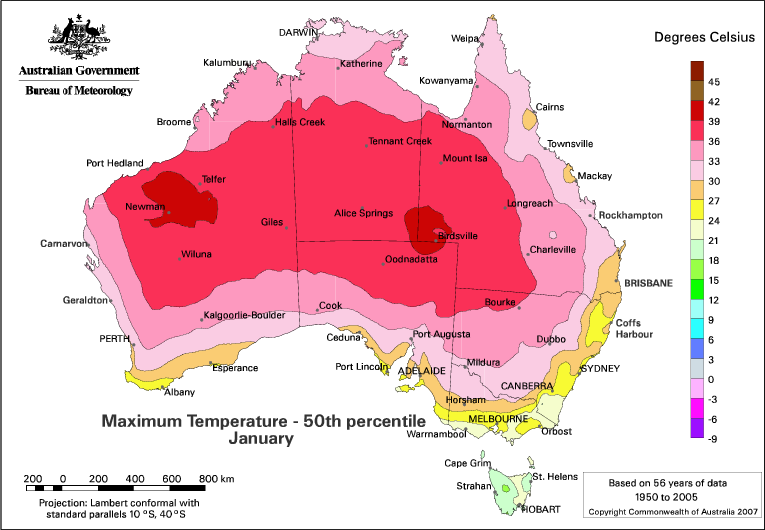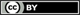# Temperature percentile maps

These temperature percentile maps show the monthly, seasonal, multi-seasonal and annual maximum, minimum and mean temperature percentile distributions across Australia.Product code: IDCJCM0010

## What do the maps show?

These maps show the monthly, seasonal, multi-seasonal and annual maximum, minimum and mean temperature percentiles for Australia. These percentiles have been calculated over the 56-year period 1950 to 2005.

For example, consider a particular location on the maximum temperature 10th percentile map for January. The temperature category (temperature value) at that location marks the threshold for the lowest 10% of records. In other words, 10% of the January maximum temperature averages recorded at this location over the period 1950 to 2005 have been cooler than the value shown on the map. Similarly, a particular location on the maximum temperature 90th percentile map marks the threshold of the highest 10% of records. This means that, at this location, 10% of the records are warmer than the maximum temperature value shown on the map.

Using these percentiles you can work out the likely occurrence of a particular event. If you have a temperature value in the 30th percentile, this means that there is roughly a 30% chance of any temperature value for that site being equal to or below this value. Similarly, if you have a temperature value in the 80th percentile, there is roughly an 80% chance that any temperature value for this site will be equal to or below this value.

Highest and lowest percentile maps give us an idea of the extremes experienced in various regions and tell us how variable the temperature can be.

These maps show the average distribution of temperature percentiles at different times across Australia. Looking at these maps, you will notice that the distribution of temperature percentiles is similar to the distribution of average temperature.

If you compare increasing temperature percentile maps, you will notice that the temperature values get higher, while still retaining a similar temperature distribution pattern.

## How are the values calculated?

Percentiles are used as an indicator of the likelihood of a particular event. Percentiles are related to deciles and are expressed as a number out of 100 (similar to a percentage). The percentile refers to the ranking of a particular value relative to all of the values for that site. For example, if there were 100 temperature values recorded for a site, we could place them in order from the coolest to the warmest temperature. Of these values, the 10th lowest value would be called the 10th percentile and the 20th lowest value the 20th percentile. This is applied right up until the 100th percentile, which includes all of the values that have been measured for that site, and is equal to the highest temperature value.Unless otherwise noted, all maps, graphs and diagrams in this page are licensed under the Creative Commons Attribution 4.0 International Licence